## MYSQL 一个SQL语句问题

xinzaibing 发布于 2012/07/13 15:30

DELETE FROM TABLE_1 A

WHERE A.PERIOD < ABS(SELECT SYSDATE-B.TIME)

FROM TABLE_1 B

WHERE A.ID1=B.ID1 AND A.ID2=B.ID2)*24*60*60;

MYSQL 5.5 下无法执行，报语法错误。

TABLE_1:   ID1 INT;

ID2 INT;

PERIOD INT;

TIME DATETIME;

0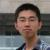select sysdate - b.time from table_1 as b where a.id1 = b.id1 and a.id2 = b.id2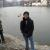1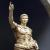To be frank: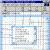@宏哥 不要再宣传更换数据库了。 问题就是问题。 虽然更换是解决问题的一种方式， 可问题是 MYSQL 能不能做这些。 不能因为 它功能弱就不解决它所遇见的问题啊。0DELETE FROM TABLE_1 A

WHERE A.PERIOD < ABS((SELECT SYSDATE-B.TIME

FROM TABLE_1 B

WHERE A.ID1=B.ID1 AND A.ID2=B.ID2)*24*60*60);

0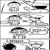#### 引用来自“xinzaibing”的答案

DELETE FROM TABLE_1 A

WHERE A.PERIOD < ABS((SELECT SYSDATE-B.TIME

FROM TABLE_1 B

WHERE A.ID1=B.ID1 AND A.ID2=B.ID2)*24*60*60);0#### 引用来自“xinzaibing”的答案

DELETE FROM TABLE_1 A

WHERE A.PERIOD < ABS((SELECT SYSDATE-B.TIME

FROM TABLE_1 B

WHERE A.ID1=B.ID1 AND A.ID2=B.ID2)*24*60*60);0#### 引用来自“xinzaibing”的答案

DELETE FROM TABLE_1 A

WHERE A.PERIOD < ABS((SELECT SYSDATE-B.TIME

FROM TABLE_1 B

WHERE A.ID1=B.ID1 AND A.ID2=B.ID2)*24*60*60);

SYSDATE-B.TIME 这个是什么。  SYSDATE 是系统关键字？还是表字段。
0